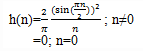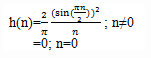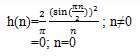Test: Hilbert Transformers Design

# Test: Hilbert Transformers Design

Test Description

## 10 Questions MCQ Test Signals and Systems | Test: Hilbert Transformers Design

Test: Hilbert Transformers Design for Electrical Engineering (EE) 2023 is part of Signals and Systems preparation. The Test: Hilbert Transformers Design questions and answers have been prepared according to the Electrical Engineering (EE) exam syllabus.The Test: Hilbert Transformers Design MCQs are made for Electrical Engineering (EE) 2023 Exam. Find important definitions, questions, notes, meanings, examples, exercises, MCQs and online tests for Test: Hilbert Transformers Design below.
Solutions of Test: Hilbert Transformers Design questions in English are available as part of our Signals and Systems for Electrical Engineering (EE) & Test: Hilbert Transformers Design solutions in Hindi for Signals and Systems course. Download more important topics, notes, lectures and mock test series for Electrical Engineering (EE) Exam by signing up for free. Attempt Test: Hilbert Transformers Design | 10 questions in 10 minutes | Mock test for Electrical Engineering (EE) preparation | Free important questions MCQ to study Signals and Systems for Electrical Engineering (EE) Exam | Download free PDF with solutions
 1 Crore+ students have signed up on EduRev. Have you?
Test: Hilbert Transformers Design - Question 1

### What kind of filter is an ideal Hilbert transformer?

Detailed Solution for Test: Hilbert Transformers Design - Question 1

Explanation: An ideal Hilbert transformer is a all pass filter.

Test: Hilbert Transformers Design - Question 2

### How much phase shift does an Hilbert transformer impart on the input?

Detailed Solution for Test: Hilbert Transformers Design - Question 2

Explanation: An ideal Hilbert transformer is a all pass filter that imparts a 90o phase shift on the signal at its input.

Test: Hilbert Transformers Design - Question 3

### In which of the following fields, Hilbert transformers are frequently used?

Detailed Solution for Test: Hilbert Transformers Design - Question 3

Explanation: Hilbert transforms are frequently used in communication systems and signal processing, as, for example, in the generation of SSB modulated signals, radar signal processing and speech signal processing.

Test: Hilbert Transformers Design - Question 4

The unit sample response of an ideal Hilbert transform isDetailed Solution for Test: Hilbert Transformers Design - Question 4

Explanation: We know that the frequency response of an ideal Hilbert transformer is given as
H(ω)= -j ;0 < ω < π
j ;-π < ω < 0
Thus the unit sample response of an ideal Hilbert transform is obtained asTest: Hilbert Transformers Design - Question 5

The unit sample response of Hilbert transform is infinite in duration and causal.

Detailed Solution for Test: Hilbert Transformers Design - Question 5

Explanation: We know that the unit sample response of the Hilbert transform is given asit sample response of an ideal Hilbert transform is infinite in duration and non-causal.

Test: Hilbert Transformers Design - Question 6

The unit sample response of Hilbert transform is:

Detailed Solution for Test: Hilbert Transformers Design - Question 6

Explanation: We know that the unit sample response of the Hilbert transform is given asThus from the above equation, we can tell that h(n)=-h(-n). Thus the unit sample response of Hilbert transform is anti-symmetric in nature.

Test: Hilbert Transformers Design - Question 7

In this section, we confine our attention on the design of FIR Hilbert transformers with h(n)=-h(M-1-n).

Detailed Solution for Test: Hilbert Transformers Design - Question 7

Explanation: In view of the fact that the ideal Hilbert transformer has an anti-symmetric unit sample response, we shall confine our attention to FIR designs in which h(n)=-h(M-1-n).

Test: Hilbert Transformers Design - Question 8

Which of the following is true regarding the frequency response of Hilbert transform?

Detailed Solution for Test: Hilbert Transformers Design - Question 8

Explanation: Our choice of an anti-symmetric unit sample response is consistent with having a purely imaginary frequency response characteristic.

Test: Hilbert Transformers Design - Question 9

It is impossible to design an all-pass digital Hilbert transformer.

Detailed Solution for Test: Hilbert Transformers Design - Question 9

Explanation: We know that when h(n) is anti-symmetric, the real valued frequency response characteristic is zero at ω=0 for both M odd and even and at ω=π when M is odd. Clearly, then, it is impossible to design an all-pass digital Hilbert transformer.

Test: Hilbert Transformers Design - Question 10

If fl and fu are the cutoff frequencies, then what is the desired real valued frequency response of a Hilbert transform filter in the frequency range 2π fl < ω < 2πfu

Detailed Solution for Test: Hilbert Transformers Design - Question 10

Explanation: The bandwidth of Hilbert transformer need only cover the bandwidth of the signal to be phase shifted. Consequently, we specify the desired real valued frequency response of a Hilbert transformer filter is
H(ω)=1; 2π fl < ω < 2πfu
where fl and fu are the cutoff frequencies.

## Signals and Systems

32 videos|76 docs|63 tests
 Use Code STAYHOME200 and get INR 200 additional OFF Use Coupon Code
Information about Test: Hilbert Transformers Design Page
In this test you can find the Exam questions for Test: Hilbert Transformers Design solved & explained in the simplest way possible. Besides giving Questions and answers for Test: Hilbert Transformers Design, EduRev gives you an ample number of Online tests for practice

## Signals and Systems

32 videos|76 docs|63 tests